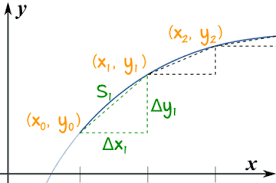## Tuesday, January 24, 2017

### Unizor - Derivatives - Differential Along a Curve

Notes to a video lecture on http://www.unizor.com

Differential Along the Curve

We will discuss curves on a plane defined parametrically as a pair of functions {x(t), y(t)}, where t is a parameter taking real values in some finite interval [a,b], while x(t) and y(t) are coordinates of points on a curve.
We assume that both coordinate functions are smooth functions of parameter t.

Our main task is to discuss the length that a point covers when it moves along this curve as parameter t changes its value within its domain.

Traditional approach to this task is to approximate the curve with a series of connected segments as presented on this picture.The idea is to increase the number of these segments, while the length of each would become smaller, and consider the sum of the lengths of these segments to approximate the length of a curve. This approximation would be better if all segments are getting smaller, so we can assume that, if there is a limit of the sum of lengths of these segments, if they are infinitesimally small, this limit is, by definition, the length of a curve.

Let's assume that we deal with a motion of a particle along this curve. It's position {x(t), y(t)} is a function of time t and the length covered by this particle during its motion along the curve would be a function of time s(t).

During the time from tn to tn+1a particle moves along the curve from point {x(tn), y(tn)} to {x(tn+1), y(t+11)}.
If all Δtn+1=tn+1−tn are sufficiently small, the length along the curve between each pair of beginning and ending points can be approximated by the length of straight segment between them. The smaller the time intervals - the better is our approximation.

So, it's reasonable to assume that if the time increment is an infinitesimal variable, the difference between exact length along the curve and the length of a straight segment is an infinitesimal of a higher order, which in subsequent summation of all infinitesimals to a total length of a curve would not affect the limit.

Let Δx(tn+1)=x(tn+1)−x(tn) and Δy(tn+1)=y(tn+1)−y(tn).
Then the length of the straight segment between two points on a curve is
Δs(tn)=Δ²x(tn)+Δ²y(tn)

As customary, with all Δtconverging to zero, we will use an infinitesimal variable dt to designate this increment of a parameter t.
With it, both Δx(tn) and Δy(tn)converge to zero and can be represented by infinitesimal variable increments dx and dy.
Analogously, increment of the length Δs(tn) is an infinitesimal variable ds.

So, the formula for infinitesimal increment of the length of a curve, called a differential along the curve, looks like this:
ds(t) = √[dx(t)]²+[dy(t)

As we know, differentials dx(t)and dy(t) can be represented as
dx(t) = x'(t)·dt and
dy(t) = y'(t)·dt
That results in the following representation of a differential along the curve:
ds(t) = √[x'(t)]²+[y'(t) ·dt

Connection to Physics

In Physics of motion we know a concept of velocity - a vector with absolute value of a ratio between distance and time (speed), directed towards the direction of motion.

If our motion is not straight forward, but goes along some curve, the direction of motion at any time is along the tangential line to this curve.
If our motion is not constant in speed, we can talk about a very small interval of time during which we can assume the speed changes just a little and calculate an average speed during this time.

Approaching this from a more mathematical viewpoint, we consider a moment of time t, a distance covered by that time as a function s(t) and infinitesimal increment of time dt.
During this infinitesimal time increment of the distance covered would be
s(t+dt) − s(t) = ds(t) =
= √[x'(t)]²+[y'(t) ·dt

Then the average speed during this infinitesimal period of time dt equals to
|V(t)| = ds(t)/dt =
= √[x'(t)]²+[y'(t)

Derivative x'(t) represents the rate of change of X-coordinate of a particle moving along the curve, derivative y'(t) is the same for Y-coordinate.
What the formula for speed along a curve shows is that velocity of an object moving along a curve, as a vector, can be represented as a sum of two vectors - X-component, a vector directed along the X-axis having value Vx(t)=x(t)=x'(t)and Y-component, a vector directed along the Y-axis having value Vy(t)=y'(t).

In vector form it looks like
V(t) = Vx(t) + Vy(t)
where overline indicates that we deal with vectors and sign + means vector addition. Here V(t) is a velocity vector, Vx(t) is its X-component - projection of velocity onto X-axis and Vy(t)is its Y-component - projection of velocity onto Y-axis.